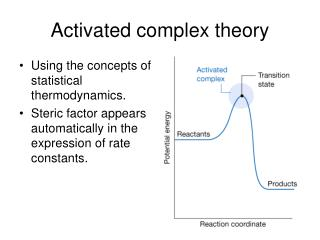DownloadDownload PresentationActivated complex theory

# Activated complex theory

Télécharger la présentation## Activated complex theory

- - - - - - - - - - - - - - - - - - - - - - - - - - - E N D - - - - - - - - - - - - - - - - - - - - - - - - - - -
##### Presentation Transcript

1. Activated complex theory • Using the concepts of statistical thermodynamics. • Steric factor appears automatically in the expression of rate constants.

2. 27.4 The Eyring equation • The transition state theory pictures a reaction between A and B as proceeding through the formation of an activated complex in a pre-equilibrium: A + B -> C‡ K‡ = ( `‡` is represented by `±` in the math style) • The partial pressure and the molar concentration has the following relationship: pJ = RT[J] • thus [C‡] = K‡ [A][B] • The activated complex falls apart by unimolecular decay into products, P, C‡ → P v = k‡[C‡] • So v = k‡ K‡ [A][B] Define k2 = k‡ K‡ v = k2[A][B]

3. (a) The rate of decay of the activated complex k‡ = κv where κ is the transmission coefficient. κ is assumed to be about 1 in the absence of information to the contrary. v is the frequency of the vibration-like motion along the reaction-coordinate.

4. (b) The concentration of the activated complex Based on Equation 20.54, we have with ∆E0 = E0(C‡) - E0(A) - E0(B) are the standard molar partition functions. provided hv/kT << 1, the above partition function can be simplified to Therefore we can write qC‡ ≈ where denotes the partition function for all the other modes of the complex. K‡ =

5. (c) The rate constant combine all the parts together, one gets k2 = k‡ K‡ = κv then we get k2 = κ (Eyring equation)

6. (d) The collisions of structureless particles A + B → AB Because A and B are structureless atoms, the only contribution to their partition functions are the translational terms: k2 = κ k2 = κ NA

7. Kinetics Salt Effect Ionic reaction A + B → C‡ C‡→ P d[P]/dt = k‡[C‡] the thermodynamic equilibrium constant Then d[P]/dt = k2[A][B] Assuming is the rate constant when the activity coefficients are 1 ( ) Debye-Huckle limiting law with A = 0.509 log(k2) = log( ) + 2AZAZBI1/2 (Analyze this equation)

8. Experimental tests of the kinetic salt effect

9. Example: The rate constant for the base hydrolysis of [CoBr(NH3)5]2+ varies with ionic strength as tabulated below. What cab be deduced about the charge of the activated complex in the rate-determining stage? I 0.0050 0.0100 0.0150 0.0200 0.0250 0.0300 k/ko 0.718 0.631 0.562 0.515 0.475 0.447 Solution: I1/2 0.071 0.100 0.122 0.141 0.158 0.173 Log(k/ko) -0.14 -0.20 -0.25 -0.29 -0.32 -0.35

10. 27.6 Reaction Collisions • Properties of incoming molecules can be controlled: 1. Translational energy. 2. Vibration energy. 3. Different orientations. • The detection of product molecules: 1. Angular distribution of products. 2. Energy distribution in the product.

11. 27.7 Potential energy surface • Can be constructed from experimental measurements or from Molecular Orbital calculations, semi-empirical methods,……

12. Potential energy surfaces, pt. 2.

13. Various trajectories through the potential energy surface

14. 27.8 Results from experiments and calculations (a) The direction of the attack and separation

15. Attractive and repulsive surfaces

16. Classical trajectories